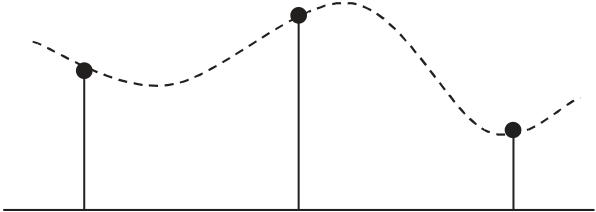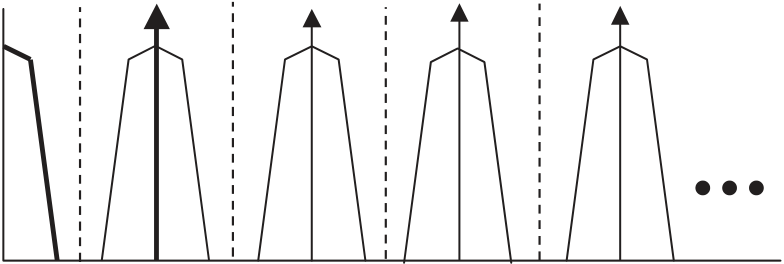Rating : ⭐⭐⭐⭐⭐
Price : \$10.99
Language:EN
Pages: 2

# The top diagram shows the original signal

724

Chapter 17

1 t fa fs fs � fa
f
fs 2M
fs fs f
fs 2M M

Figure 17.115a shows how to decimate the output of an FIR fi lter. The fi ltered data y(n) is stored in a data register that is clocked at the decimated frequency f s /M. This does not change the number of computations required of the digital fi lter; i.e., it still must calculate each output sample y(n).

Figure 17.115b shows a method for increasing the computational effi ciency of the FIR fi lter by a factor of M. The data from the delay registers are simply stored in N data registers that

www.newnespress.com
Filter Design
x(n) z�1 h(1)
z�1
z�1

Σ

y(n) Clock =

fs
M

h(0) h(2) h(N�1) Data y(m) ↓ M
h(0) z�1 h(1) z�1 z�1
Clock =

fs

y(m) ↓ M

h(N�1)
h(2)
(b)
Σ

are clocked at the decimated frequency f s /M. The FIR multiply-accumulates now only have to be done every Mth clock cycle. This increase in effi ciency could be utilized by adding more taps to the FIR fi lter, doing other computations in the extra time, or using a slower DSP.

Figure 17.116 shows the concept of interpolation. The original signal in 17.116a is sampled at a frequency f s . In 17.116b, the sampling frequency has been increased by a factor of L, and zeros have been added to fi ll in the extra samples. The signal with added zeros is passed through an interpolation fi lter, which provides the extra data values.

www.newnespress.com
How It Works